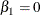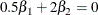# The MDC Procedure

### TEST Statement

<'label':>

• TEST <'string':> equation <,equation…> </ options> ;

The TEST statement performs Wald, Lagrange multiplier, and likelihood ratio tests of linear hypotheses about the regression parameters in the preceding MODEL statement. Each equation specifies a linear hypothesis to be tested. All hypotheses in one TEST statement are tested jointly. Variable names in the equations must correspond to regressors in the preceding MODEL statement, and each name represents the coefficient of the corresponding regressor. The keyword INTERCEPT refers to the coefficient of the intercept.

The following options can be specified after the slash (/):

ALL

requests Wald, Lagrange multiplier, and likelihood ratio tests.

WALD

requests the Wald test.

LM

requests the Lagrange multiplier test.

LR

requests the likelihood ratio test.

The following statements illustrate the use of the TEST statement:

   proc mdc;
model decision = x1 x2 / type=clogit
choice=(mode 1 2 3);
id pid;
test x1 = 0, 0.5 * x1 + 2 * x2 = 0;
run;


The test investigates the joint hypothesis thatandOnly linear equality restrictions and tests are permitted in PROC MDC. Tests expressions can be composed only of algebraic operations that use the addition symbol (+), subtraction symbol (–), and multiplication symbol (*).

The TEST statement accepts labels that are reproduced in the printed output. The TEST statement can be labeled in two ways. A TEST statement can be preceded by a label followed by a colon. Alternatively, the keyword TEST can be followed by a quoted string followed by a colon. If both are present, PROC MDC uses the label that precedes the first colon. If no label is present, PROC MDC automatically labels the tests.